Category: Blog

# PySLM: Geometric Hatch Overlap Check/Visualisation

Designing scan strategies for PBF techniques, we are not entirely aware of situations that arise where the powder-bed is not fully exposed due to a mismatch when scan vectors are not sufficiently overlapped. Typically, unoptimised placement of hatch vectors lead to the creation of irregular porosity or voids in L-PBF parts.

This can arise along the intersections between the contour and interior hatches, especially a long concave regions such as sharp corner features with acute angles.

The approach is not an efficient way to examine the presence of , but provides a representative view for checking this geometrically.

## Method

The approach takes advantage of the relatively new Iterator classes available within the analysis module, which vastly simplifies the generation procedure for manipulating and examining existing scan vector geometry. Firstly, generate or alternatively import the Layer and its LayerGeometry groups to examine. The group of layers are passed to the ScanVectorIterator class, which will iterate across every scan vector from both ContourGeometry and HatchGeometry objects within a Layer. Single point exposures are not considered.

import pyslm.analysis
from shapely.geometry import LineString, Polygon, MultiPolygon

scanIterator =  pyslm.analysis.ScanVectorIterator([layer])


After the creation of the ScanVectorIterator, this can be readily expedited to process across all scan vectors across the Layer. The basic process relies on converting each scan vector to a Shapely polygon objects and then processing them using the geometry tools available.

For this case we use Pythonic notation to compactly operate across each scan vector and collect them. We convert each scan vector to a Shapely LineString, which has a method to then offset or buffer.

# Laser Spot Radius

# Iterate across each scan vector and buffer than geometry
lines = [LineString(line).buffer(laserSpotRadius) for line in scanIterator]


After offseting all the lines, this can be easily visualised by conversion to a Shapely MultiPolygon.

# Merged the offset lines into a Shapely Multi-Polygon Collection
multiPoly = MultiPolygon(lines)
pyslm.visualise.plotPolygon(multiPoly)

The geometrical result is shown below. It is relatively quick to generate and plot individual scan vectors as shown below:

Each scan vector that is offset is represented by a Shapely.Polygon. It is trivial to perform a boolean operation with the Shapely library. Although, it is recommended to use the more efficient, albeit still relatively slow, shapely.ops.cascaded_union function to merge multiple geometries together:

# Cascaded union is a more efficient boolean merge for multiple polygon entities

pyslm.visualise.plotPolygon(multiPolyMerged)

The combined result is shown here with a slightly smaller hatch distance to exaggerate the effect and highlight regions where the laser beam may not sufficiently provide exposure to the powder bed: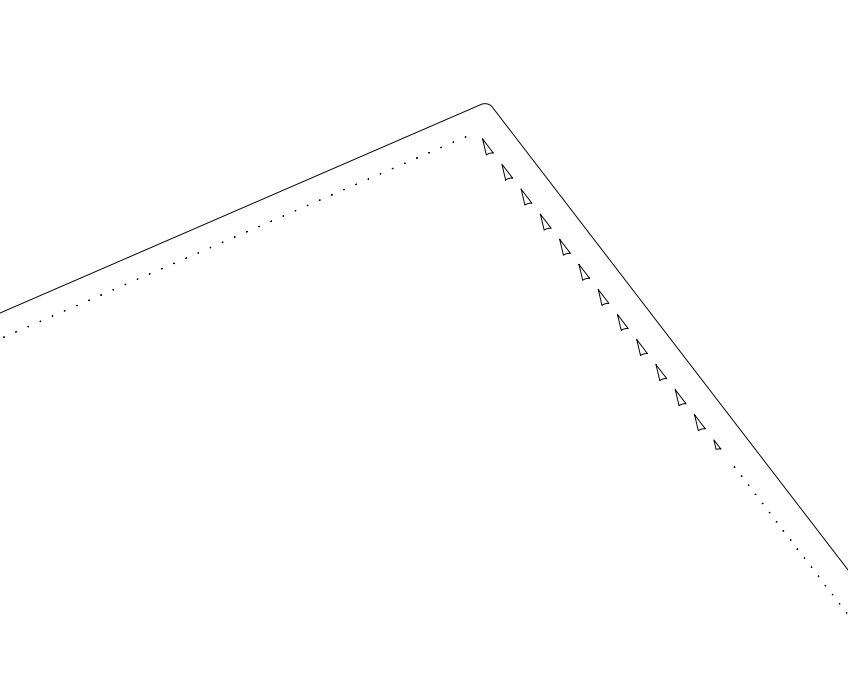Regions that have insufficient coverage observed after performing a boolean merge after the scan vectors have been offset

This post shares a relatively simple example exploration using geometrical operations and the iterator class to understand potential issues related to hatch overlaps.

## Final Conclusions

Potentially, this check could be extended into 3D using morphological operations. This would provide a more qualitative examination of porosity generation as a result of Furthermore, the combined use of Neural Networks or reduce order models could provide a representative exposure area to provide the geometrical offset in 2D and provide a prediction for coverage spatially in 3D.

# PySLM 0.4

PySLM is a more frequent update to the library with few additions, due to mainly resolving issues fixing several bugs including suggestions and feedback from several users along the way. I wish to thank them personally for their support along the way, as it does help improve the PySLM library for everyone.

The release primarily includes a new analysis Iterator feature that provides a set of classes for iterating across the layer geometry definitions. This builds upon previous work in the previous version of the c++ libSLM, however, for reference been implemented in Python.This includes iterating across individual LayerGeometry regions via LayerGeometryIterator and Scan Vectors via ScanvVectorIterator. Useful for simulation and numerical studies is the ScanIterator class that aims to replicate the spatial position and laser parameters across time given the laser input. This is particularly useful for numerical simulations for predicting the thermal and thermo-mechanical behaviour during the process. The exposure points generated in ScanIterator class are based on a timestep, that can be controlled directly via the analysis or exported to a .csv file for use by an external program. The infrastructure behind the iterators was derived for efficient generation and computation using a tree structure for caching data.

Another nice feature of note is the correct visualisation of scan paths based on their true scanning order across both hatch and contour scan geometry using the new visualise function plotSequential as described in detail in a previous post. This includes a nicer visualisation of jump vectors that was previous missing.

Another example for generating parametric studies is included to support research on the development of process parameters for new materials on machine systems, in conjunction with DOE tools available in python.

Once again the support module has been put on hold, whilst investigating and researching a more efficient approach for isolating support regions and generating their assigned support geometry regions.

The release finally marks a small achievement in getting an automatic build system in place used Github Actions for compiling and publishing the python packages to PyPi repository, for both most versions (3.5-3.9) of Python on both Windows and Mac OS X.

Finally, the complete release log may be found on github at pyslm/CHANGELOG.MD.

# PySLM Scan Path Iterator

## Historical Background

An upcoming key feature in PySLM is the Iterators primarily useful for simulation studies, such as predicting thermo-mechanical behavior of scan strategies. Much of this builds upon ideas in former work that was done during my PhD for investigating the generation of residual stress in selective laser melting. In that study, MSC Marc, a commercial Finite Element analysis package was used to predict residual stresses generated during the process. The discretised position and laser parameters of the exposure from the laser was controlled by combination of Fortran User Subroutines and libSLM, the former c++ library.

Prior to running, a configuration file was passed to the simulation, specifying the SLM machine build file used for simulating the scan paths. libSLM parsed the compatible build-file and then based on the current time and increment would interpolate the position of the exposure point and laser parameters during firing. Beyond this main functionality, there were additional house keeping required for running the simulation, including passing information between the programs, and also additional tools to efficiently seek at an arbitrarily point in time, the state and position of the laser. This was necessary for restarting simulations on a HPC and for adaptive time-stepping required to keep numerical stability. For efficient seeking across the layers and each layer geometry structure was cached within a tree, that could be parsed on demand if necessary. Much of the infrastructure was excessive, although the implementation had to be written in c++ or Fortran to be used by integrated with the commercial solver.

Although it is difficult to perceive the full benefit of having a Python version of the same functionality, there are some instances and some analysis codes where this could be of benefit for modelling this and other processes as well.

## Implementation of PySLM Iterators

The implementation builds upon the existing design from the original libSLM library. For all the Iterator classes, similar to most of the other pyslm.analysis module’s tools, the list of Layers and Models with the Laser Parameters should be passed:

# Iterates across layer geometries
layerGeomIter = Iterator(models, layerList)

# Iterates across individual scan vectors - currently only ContourGeometry/HatchGeometry
scanVectorIter = ScanVectorIterator(models, layerList)

# Generates an scan exposure point iterator
scanIter = ScanIterator(models, layerList)


The first stage is building a time cache tree across each LayerGeometry. In practice, the cache tree structure is not necessary if the scan iterator iteratively increments along in time. Having a cache structure enables non-linear movement of the iterator across the entire build . It also provides a fast random-access lookup to seek to a specific Layer or LayerGeometry for use in simulations or analyses.

This structure is formed by iteratively measuring the total time taken to scan an individual LayerGeometry group which is stored in a tree node (TimeNode). The cumulative time taken to scan across each LayerGeometry TimeNode to provide the total scan time across the Layer. The TimeNode can be assigned child and parent nodes using the attributes (TimeNode.parent and TimeNode.children) in order to navigate across the entire tree. Each TimeNode provides a key-value pair (id, value) to store the reference LayerGeometry or Layer for simplified access.

The Cache Tree is generated and stored in the Base Class, Iterator and is generated in the private method (Iterator._generateCache) and stored in the attribute Iterator.tree.

def _generateCache(self) -> None:
self._tree = TimeNode() for layerId, layer in enumerate(self.layers):     # Create the layer     layerNode = TimeNode(self._tree, id=layerId, value=layer)     self._tree.children.append(layerNode)     for layerGeomId, layerGeom in enumerate(layer.geometry):         geomNode = TimeNode(layerNode, id=layerGeomId, value=layerGeom)         geomNode.time = getLayerGeometryTime(layerGeom, self._models)         layerNode.children.append(geomNode) self._cacheValid = True

The Iterator class has many useful facilities, such as build-time estimation, seeking access to the Layer or LayerGeometry at an arbitrary point in time. The class stores additional info such as the layer dwellTime – this can be re-implemented in a derived class. For implementing the iterator behavior used across all dependent classes it also stores the current time and reference pointers to the current Layer and LayerGeometry. Essentially the Iterator class can be used to iterate across each LayerGeometry within a build as a foundation to the other class. Each of these Iterator classes builds upon the magic methods available in Python: __iter__ and __next__ . The __iter__ method simply sets up the object and re-initialises the Iterators attributes. Once the cache tree is generated internally, it offers no penalty to generate a new iterator . Below is an excerpt taken from the ScanVectorIterator:

def __iter__(self):
self._time = 0.0
self._layerGeomTime = 0.0
self._layerInc = 0
self._layerGeomInc = 0

return self

def __next__(self):
if self._layerScanVecIt < len( self._layerScanVectors):
scanVector = self._layerScanVectors[self._layerScanVecIt]
self._layerScanVecIt += 1
return scanVector
else:
# New layer
if self._layerIt < len(self._layers):
layerVectors = self.getLayerVectors(self._layers[self._layerIt])
self._layerScanVectors = self.reshapeVectors(layerVectors)
self._layerScanVecIt = 0
self._layerIt += 1
return self.next()
else:
raise StopIteration

The Iterator class and ScanVectorIterator class do not require much further attention, as the pointer to the geometry is incremented only. The ScanIterator class, however, is more useful for simulation and will be discussed further.

#### Scan Iterator Class

The ScanIterator class is used for incrementally advancing the exposure source across each scan vector. This is particularly important for visualising or simulating the AM process. The time increment is based on a chosen but adjustable timestep, and the laser parameters across each scan vector (i.e. the effective scan velocity) obtained from the assigned BuildStyle.

The exposure point is linearly interpolated across each scan vector based on the current time within the LayerGeometry depending on the type. For identifying the position, the cumulative distance is captured and the current timeOffset for the layer geometry is used to estimate the distance covered by the exposure source across the entire LayerGeometry section. For simplicity this assumes no acceleration terms and uses a constant velocity profile. Based on the timeOffset, the scan vector is obtained and then the final position is interpolated across the scan vector.

laserVelocity = getEffectiveLaserSpeed(buildStyle)

# Find the cumulative distance across the scan vectors in the LayerGeometry (Contour)
delta = np.diff(layerGeom.coords, axis=0)
dist = np.hypot(delta[:,0], delta[:,1])
cumDist = np.cumsum(dist)
cumDist2 = np.insert(cumDist, 0,0)

# If the offsetDist calculated is outside of the cumulative distance then some error has occured
if offsetDist > cumDist2[-1]:
raise Exception('Error offset distance > cumDist {:.3f}, {:.3f}'.format(offsetDist, cumDist2[-1]))

id = 0

# Find the id of the current scan vector given the time offset
for i, vec in enumerate(cumDist2):
if offsetDist < vec:
id = i
break

# interpolate the position based on offset distance in the scan vector
linearOffset = (offsetDist - cumDist2[id-1]) / dist[id-1]
point = layerGeom.coords[id-1] + delta[id-1] * linearOffset

The above example is specifically for the contour geometry. Note the for loop is not particularly efficient but serves its purpose for identifying the Iterator’s current scan vector.

### Iterator Use:

Each iterator can be subsequently called after using the iter method in a variety of pythonic ways:

#Create a scan vector iterator
ScanVectorIterator(models, layerList)

# Create a python iter object from a ScanVectorIterator
scanIter = iter(scanVectorIter)

# Get a single scan vector
firstScanVec = more(scanIter)

# Collect all the remaining scan vectors
scanVectors = np.array([point for point in scanIter])

### Current Limitations:

Note the current implementation of the iterators currently only consider ContourGeometry and HatchGeometry and does not include PointGeometry groups. The jump vectors are ignored, which will have a small but in most situations a negligible effect on the the overall accuracy of the timing used for the iterators.

Another obvious limitation is that this only accounts for single exposure source systems. It is not known to myself, how multiple-exposure systems scan (i.e. are they truly in parallel based on the laser number) or is there is some built-in machine heuristic which balances the scanning across all laser sources and spatially – e.g. to prevent overheating. This depends on the SLM system such as if multiple exposure sources are limited by zones or have full areal access to the bed. Anyone’s comments or experiences on this aspect would be sincerely welcomed.

### Example

An example showing the basic usage and functions available with the Iterator classes are available in the Github Repo examples/example_laser_iterator.py

# Finding Overhangs in 3D Printing using PySLM

Determining overhang regions are crucial for detected potential failure points for unsupported regions that are necessary to generate support structures for 3D Printing. Typically, for triangular meshes, this is calculated by taking the dot-product between the triangle normal and the vertical build direction and collecting the values across the entire mesh.

v0 = np.array([[0., 0., -1.0]])
v1 = part.geometry.face_normals
theta = np.arccos(np.clip(np.dot(v0, v1.T), -1.0, 1.0))
theta = np.degrees(theta).flatten()

The approach is not particularly complicated. However, depending on some geometries, especially those from topology optimised geometries tend to be have ‘noise’ in the surface triangles, so patches can appear within areas generally considering to require support.A close-up of an overhang angle showing ‘noisy’ disconnected regions. This is a result of the surfaces slightly above the overhang angle tolerance despite neighbors being under.

One approach taken is using the surface connectivity information to smooth or average the surface normal or overhang angle in order to reduce these discontinuities. The approach taken gathers the adjacent triangle faces and uses the information to identify the overhang angle. This option is enabled when passing the useConnectivity=true argument in pyslm.support.getOverhangMesh (will become available at a later date).

The approach uses the face adjacency information built-into Trimesh’s meshes and collects this in order to map the connectivity between faces. The list of adjacent faces are stored as a Python dict so that they can mapped on-demand.

def getAdjacentFaces(part: Part):
mesh = part.geometry

import networkx as nx

graph = nx.Graph()

adjacentFaces = {node: list(graph.neighbors(node)) for node in graph.nodes}
return adjacentFaces

Once the facial connectivity is found, each face within the mesh is iterated over. The average overhang angle is calculated accordingly by collecting the faces from the adjacency list into conFaces and then using this to index the numpy array directly.

theta = np.degrees(theta).flatten()
thetaAvg = theta.copy()
thetaAvg[face] = np.mean(theta[conFaces])

The difference is highlighted below.

### Ray Projection Investigation

The support generation – described in this post, builds upon ray projection or ‘ray casting’ method to identify intersecting support areas. The idea was looking at how one could identify supports that are only in contact with the base/build plate – typically acceptable. For reasons later explained, it can be better to work beyond the overhang regions identified based on just the triangular meshes because they can have jagged edges, even with the connectivity approach. It it also not trivial to interpolate across the surface or use polygon offsetting algorithms.

The idea is simple, rays are uniformly distributed and projected underneath the mesh to identify the intercepts with the mesh to find the distance from the build plate. The polygon of the bounding box is generated from the mesh and then is discretised with points using the rasterize function for trimesh.path.Path2D. The rays are projecting using Trimesh’s in-built ray projection facility from the seed points (note: the PyEmbree wrapper library as an auxiliary method does not provide enough accuracy to do this).

# Generate a polygon covering the part's bounding box

# Rasterise the surface of overhang to generate projection points
supportArea = np.array(offsetPoly.rasterize(resolution, offsetPoly.bounds[0, :])).T

coords = np.argwhere(supportArea).astype(np.float32) * resolution

# Project upwards to intersect with the upper surface
# Set the z-coordinates for the ray origin
coords = np.insert(coords, 2, values=-1e5, axis=1)
rays = np.repeat([upVec], coords.shape, axis=0)

#Find the first location of any triangles which intersect with the part
hitLoc, index_ray, index_tri = mesh.ray.intersects_location(ray_origins=coords, ray_directions=rays,multiple_hits=False)
print('\t - finished projecting rays')

The first hit locations with the mesh are stored and the heights are then transformed back to a height-map based on the original bounding-box and resolution used.

heightMap = np.ones(supportArea.shape) * -1.0
if len(hitLoc) > 0:
hitLocCpy = hitLoc.copy()
hitLocCpy[:, :2] -= offsetPoly.bounds[0, :]
hitLocCpy[:, :2] /= resolution
hitLocIdx = np.ceil(hitLocCpy[:, :2]).astype(np.int32) # Assign the heights heightMap[hitLocIdx[:, 0], hitLocIdx[:, 1]] = hitLoc[:, 2]

From the height map, the gradients are calculated using 1st order difference stencil within Numpy’s np.gradient function, along each direction. The magnitude is found and the overhang angle is calculated based on the resolution.

gradX = np.gradient(heightMapFix, axis=1)
angle = np.degrees(np.arctan(mag/resolution))

Once the angle is obtained the projected overhang regions can be found using the Marching Square algorithm built into Scikit Image (skimage.measure.find_contours). The isolevel chosen is based on the overhang angle and the resolution chosen alongside some carefully selected fudge-factor. A mask is used to remove areas in the background (non-hit areas) based on the background

isolevel = 1.1 * np.tan(np.deg2rad(overhangAngle)) * resolution
contours = skimage.measure.find_contours(mag,isolevel, mask=heightMapFix>1e-3)

The result can be seen below.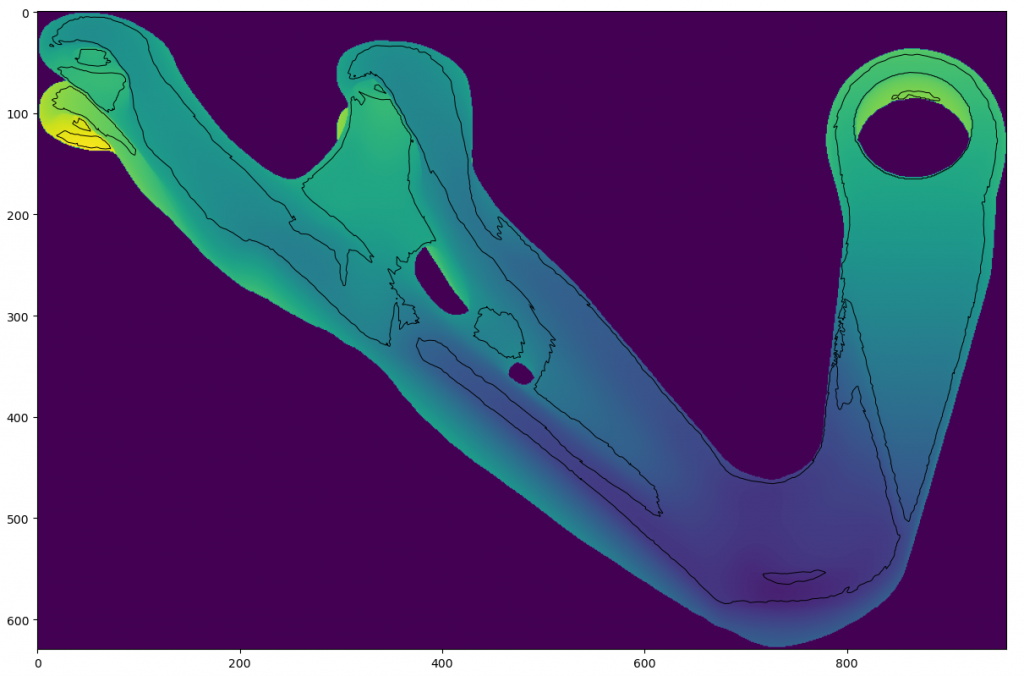The projected overhang (45°) region for a topology optimised bracket overlaid on the height map found using ray projection

The benefits of this approach are not immediately apparent. However, the contour information can be used and smoothed when projecting supports back onto the part. The limitation with the approach is that the projection’s resolution is determined by the overhang angle captured and obviously is based on the line of sight – so any self-intersecting supports with the part are neglected. The approach can be further explored using GLSL shaders in order to remove the bottleneck of ray tracing and achieve the same result.

This method is included as a utility function (pyslm.support.generateHeightMap) and both functions will be made later available with the introduction of the support module.

# PySLM: Layer Visualisation Update

Following a recent suggestion, it was useful to revisit the basic visualisation functionality within PySLM for visualising LayerGeometry in a Layer. The request suggested was related to displaying jump vectors between vectors and implementing this within the visualisation. Jump vectors visualise, the jump across adjacent scan vectors when the laser or energy source is not firing . Although it is a trivial matter, good visualisation helps us to understand how scan vectors are processed by the additive manufacturing machine.

The current implementation does visualise the order of scanning but not correctly in its previous form. All HatchGeometry and ContourGeometry is gathered across the layer and processed separately. The order of scanning within the HatchGeometry is found by collecting across all the scan vectors and simply assigning a range across all scan vectors, using numpy.arange.

# Plot the sequential index of the hatch vector
lc.set_array(np.arange(len(hatches)))

Obviously, the current approach isolates the hatch and contour scan vectors so even when the contour vectors are scanned first or there is an arbitrary order of scanning across types – especially for complex scan strategy arrangements, the visualisation is incorrect. Likewise, for displaying the jump vectors, this would not work correctly.

### New Approach

Restarting, the approach simply takes existing collection of LayerGeometry and then gathers together all the vectors across both HatchGeometry and ContourGeometry object. However, ContourGeometry vectors are joined together by coordinates rather than discrete pair of coordinates representing the vectors. Across the ContourGeometry, the vectors are transformed into the equivalent hatch vectors in order to be consistent when using Matplotlib’s LineCollection objects. This is done by stacking the ContourGeometry’s coordinates by offsetting by a single coordinate position.

scanVectors = []
for geom in layer.geometry:

if isinstance(geom, HatchGeometry):
coords = geom.coords.reshape(-1, 2, 2)
elif isinstance(geom, ContourGeometry):
coords = np.hstack([geom.coords, np.roll(geom.coords, -1, axis=0)]).reshape(-1,2,2)

scanVectors.append(coords)

scanVectors = np.vstack(scanVectors)

These can then be plotted using Matplotlib LineCollection:

lc = matplotlib.collections.LineCollection(scanVectors, cmap=plt.cm.rainbow, linewidths=1.0)

Unfortunately, there is some inefficiency merging all the scan vectors due to copy operations and stacking the array structure, although for the purpose of visualising single layers it is satisfactory.

Depending on the geometry, especially those with many facets in the mesh, the slicing results in contours which contain many edges, that may persist even after polygonal simplification. For representing the scan order by changing the colour hue, this may result in colour map biased with the contours when attempting to visualise the order.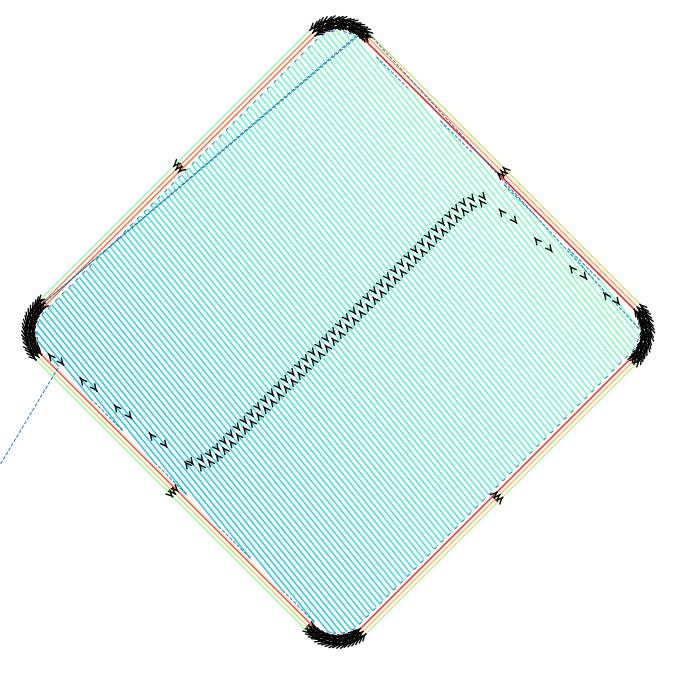The range of colour are limited across all the scan vectors due to the large number of edges on the outer border

A method to circumvent this is to use the cumulative distance across each scan vector that is taken across the entire layer. This acts as a way to normalise the scan order irrespective of complexity, length and distribution of scan vectors across the entire length.

delta = scanVectors[:, 1, :] - scanVectors[:, 0, :]
dist = np.sqrt(delta[:, 0] * delta[:, 0] + delta[:, 1] * delta[:, 1])
cumDist = np.cumsum(dist)
lc.set_array(cumDist.ravel())

The final step is accounting for the jump vectors i.e. when the galvo mirrors move between scan vectors without the energy source activated. Having a consistent list of scan vectors means this is straightforward to calculate by replicating the same technique used for translating the ContourGeometry into hatches:

scanVectors = np.vstack(scanVectors)
svTmp = scanVectors.copy().reshape(-1,2)
svTmp = np.roll(svTmp,-1,axis=0)[0:-2]
svTmp = svTmp.reshape(-1,2,2)

lc2 = mc.LineCollection(svTmp, cmap=plt.cm.get_cmap('Greys'), linewidths=0.3, linestyles="--", lw=0.7)

This involves taking every odd pair across all the scan vector lists. These are plotted as line collections with dashes – that are more appealing on the eye. However, this results in ‘invisible’ jump lines between the scan vectors during Contour scans. Nevertheless, it presents a simple approach to generating more informative visuals for the scan vector paths across the layer. The final result is shown below.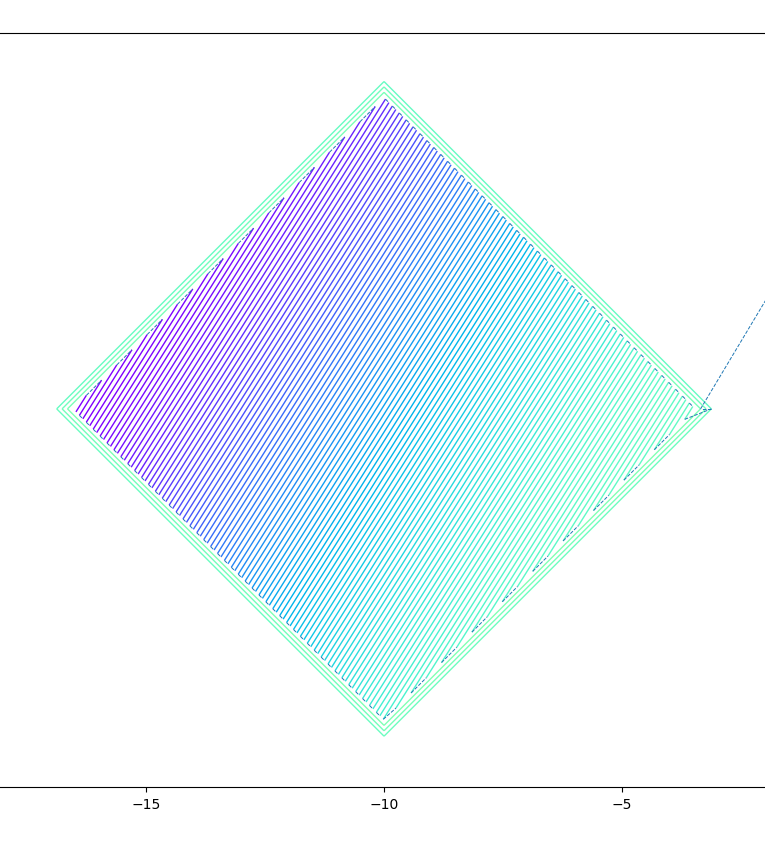New sequential visualisation showing sequential scanning of both hatch and contour geometries with jump vectors (dashed lines)

This new function for plotting can be found in pyslm.visualise.plotSequential.

# PySLM Version 0.3

PySLM version 0.3 was released last week to coincide with a large number requests from users of the library. The release is consists of many updates, fixes and examples accumulated across the last 6 months since last summer. Additional work was done to refine the release of the sister library libSLM and resolve some bugs that couldn’t be determined until exporting the machine files and testing on the machine, with acknowledgement of support from researchers who have got in touch. Many thanks for their assistance on this development journey.

The release notes can be found on github.

The original release was scheduled to include support generation, but this has been postponed for v0.4 to ensure that there was a underlying stable release of PySLM as reference to ensure users can utilise the library without waiting for the support structure element to stabilise.

A summary of notable features amongst fixes are as follows:

• Added class geometry.utils.ModelValidator with functions to validates the inputs (models, layers) are consistent and coherent prior to exporting to machine build files using libSLM.
• Added an alternative method BaseHatcher.clipContourLines for clipping a list of contour scan vectors. See the previous post about generating custom sinusoidal scan strategy using this method.
• Added method hatching.simplifyBoundaries to simplify boundaries using Sci-kit Image method based on Douglas-Peucker algorithm.
• Added a methodvisualise.visualiseOverhang to visualise overhangs – in preparation for support structure analysis
• Added function argument index to visualise.plot in order to visualise the scan vector parameters (e.g. length, laser parameters, build style id)

Any requests for additional features or other improvements feel free to get in touch.

# Custom Scan Strategies in SLM / L-PBF with PySLM: Sinusoidal Scanning

Building upon the previous post that provided a detailed breakdown for creating custom island scan strategies, this further post documents a method for deploying custom ‘hatch’ infills. This is particularly desirable capability sought by researchers and has been touched upon very little in the current research. The use of unit-cell infills or in particular fractal filling curves such as the Hilbert curve have been sought for better controlling the thermal history and melt pool stability of hatch infills.

This has been previously explored in SLS ] and in SLM on a previous collaborators at the University of Nottingham investigating Fractal scanning strategy .

Typically, hatch infills are sequences of linear lines that form the the ‘hatch’ pattern. Practically, these are very efficient mechanism for infilling a 2D area by using 1D line elements when rastering a laser. Clipping of lines within polygons is intuitive. As discussed there are various scan strategies that can be employed to generate variations on this infill – i.e. stripe, checkerboard/island scan strategy and also modifying the order or sorting of the hatch vectors.

Geometrical scan strategies that adapt the infill based on the underlying geometry, i.e. lattices are acknowledges as ways for drastically improving the performance and the quality of these characteristic structures. This would be based on some medial-axis approach. This post will not specifically delve into this, rather, demonstrate an approach for custom infills on bulk regions.

Ultimately, drastically changing the behavior of the underlying hatch infill has not really been explored. This post will demonstrate an example that could be employed and explored as part of future research.

### Custom Sinusoidal Approach

Sinusoidal scanning has been employed in welding research and also in direct energy deposition (DED) in order to improve the stability and quality of the joining or manufacturing process.

The process of generating this particular scan strategy requires some careful thought to improve the efficiency of the generation, especially given the overall increase in number of points require to essentially ‘sample’ across the sin curve.

The implementation requires subclassing the Hatcher class, by re-implementing the BaseHatcher.generateHatching and the BaseHatcher.hatch methods.

Unlike, the normal hatch vectors, the sinusoidal pattern has to be treated as a series of connected line segments, without any jumping. This requires using the ContourGeometry representation to efficiently store the discretised curve. As a result, the Hatcher.hatch method has to be re-implemented to take account of this.

The procedure builds upon previous methods to define customer behavior (see previous post). The first steps are to define a local coordinate system x' and y' for generating the individual sin curve. A sine curve y' = A \sin(k x') is generated to fill the region bounding box accordingly, given a frequency and amplitude parameter along x'.

The number of points used to discretise the sine curve is determined by \delta x. This needs to be chosen to suit the parameters for the periodicity and amplitude of the sine curve. A reasonable compromise is require as this will severely impact both the performance of clipping these curves, but also the overall file size of the build file generated.

dx = self._discretisation # num points per mm

hatches = x.copy()

"""
Generate the sinusoidal curve along the local coordinate system x' and y'. These will be later tiled and then
transformed across the entire coordinate space.
"""
yDash = self._amplitude * np.sin(2.0*np.pi * self._frequency * xDash)

"""
We replicate and transform the sine curve along adjacent paths and transform along the y-direction
"""
y = np.tile(yDash, [x.shape, 1])
y += x

x = np.tile(xDash, [x.shape,1]).flatten()
y = y.ravel()

After generating single sine curve, numpy.tile is used to efficiently replicate the curve to fill the entire bounding box region. Each curve is then translated by an increment defined by x, to represent the effective hatch spacing or hatch distance.

The next important step is to define the sort order for scanning these. This is slightly different, in that the sort order is done per line segment used to discretise the curve. This is subtle, but very important because this ensures that the curves when clipped by the slice boundary are scanned in the same prescribed sequential order.

The increment of 1\times10^5 is used in order to potentially differentiate each curve later, if required.

# Seperate the z-order index per group
inc = np.arange(0, 10000*(xDash.shape), 10000).astype(np.int64).reshape(-1,1)
zInc = np.tile(inc, [1,hatches.shape]).flatten()
z += zInc

coords = np.hstack([x.reshape(-1, 1),
y.reshape(-1, 1),
z.reshape(-1, 1)])

Following the generation of these sinusoidal curves, a transformation matrix is applied accordingly, before these are clipped in the Hatcher.hatch method.

The next crucial difference, that has been implemented from PySLM version 0.3, is a new clipping method, BaseHatcher.clipContourLines. The following method is different from BaseHatcher.clipLines, in that clips ContourGeometry separately. This is important for keeping the scan vectors separate and in the correct order, which would be otherwise difficult to achieve. The clipped results are implicitly separated into contour geometry groups.

hatches = self.generateHatching(paths, self._hatchDistance, layerHatchAngle)

clippedPaths = self.clipContourLines(paths, hatches)

# Merge the lines together
if len(clippedPaths) > 0:
for path in clippedPaths:
clippedLines = np.vstack(path)

clippedLines = clippedLines[:,:2]
contourGeom = ContourGeometry()

contourGeom.coords = clippedLines.reshape(-1, 2)

layer.geometry.append(contourGeom)

The next step is to sort the clipped paths into the right order. This is done by using the 1st value of 3rd index column accordingly sorting using sorted with a lambda function.

"""
Sort the sinusoidal vectors based on the 1st coordinate's sort id (column 3). This only sorts individual paths
rather than the contours internally.
"""
clippedPaths = sorted(clippedPaths, key=lambda x: x)


Now, the result of the sinusoidal scan strategy can be visualised below.

This approach currently is very intensive to generate during the clipping operation, due to the number of edges along each clipping operation. Using the previous techniques with the island scan strategy in a previous post, could be use to amorotise a lot of the cost of clipping.

### Example Script

The script is available on github at examples/example_custom_sinusoidal_scanning.py

References

# Custom Island Scan Strategies for L-PBF/SLM using PySLM

The fact that most island scan strategies employed in SLM are nearly always square raised the question whether we could do more. I recently came across this ability to define ‘hexagon’ island regions advertised in the 2020 release of Autodesk Netfabb. Unfortunately this is a commercial tool and not always available. The practical reasons for implementing a hexagon island scanning strategy are largely unclear, but this prompted to create an example to illustrate how one would create custom island regions using PySLM. This in future could open some interesting ideas of tuning the scan strategy spatially across a layer.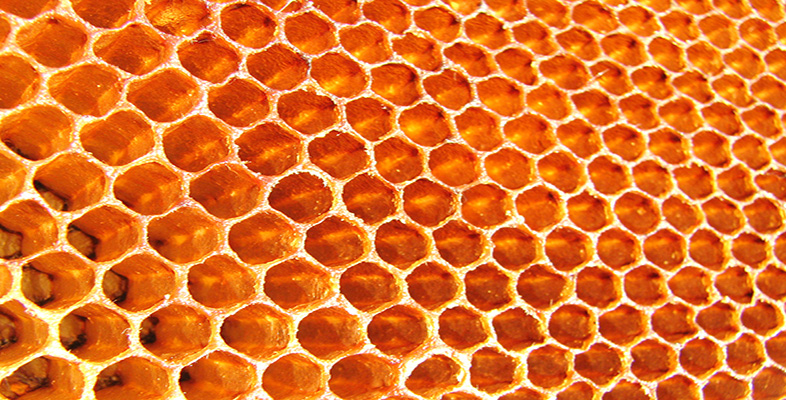Honeycombs or heaxgonal lattices observed in nature are a popular structure used in composites engineering. Could the same be applied in Additive Manufacturing?

The user needs to customise the behaviour they desire by deriving subclasses from:

• hatching.Island
• hatching.IslandHatcher

These classes serve the purpose for defining a ‘regular’ tessellated sub-region containing hatches. Regular regions that share the same shape characteristics for using the infill optimises the overall clipping performance outlined in the previous post.

Theoretically, we could build 2D unstructured cells e.g. Voronoi patterns, however, internally hatches for each region will require individual clipping and penalised with a significant performance hit during the hatching process.Example of a Voronoi diagram: regions are dibi based on the boundaries between.

The Island subclass region is the most important part to re-define the behavior. If we want to change the island regions to become regular tessellated polygons, the localBoundary method should be re-defined. In this example, it will generate a hexagon region, but the implementation below should be generic to cover other N-gon primitives:

   def localBoundary(self) -> np.ndarray:
# Redefine the local boundary to be the hexagon shape

if HexIsland._boundary is None:
# Simple approach is to use a radius to define the overall island size

numPoints = 6

radius = self._islandWidth / np.cos(np.pi/numPoints)  / 2 + self._islandOverlap

# Generate polygon island
coords = np.zeros((numPoints+1, 2))

for i in np.arange(0,numPoints):
# Subtracting -0.5 orientates the polygon along its face
angle = (i-0.5)/numPoints*2*np.pi
coords[i] = [np.cos(angle), np.sin(angle)]

# Close the polygon
coords[-1] = coords

# Scale the polygon

# Assign to the static class attribute
HexIsland._boundary = coords

return HexIsland._boundary

The polygon shape is defined by numPoints, so this can be changed to another polygon if desired. The polygon boundary is defined using a radius for the island region and from this a regular polygon is constructed on the outside. The polygon points are rotated by adjusting the start angle so there is a vertical edge on the RHS.

This is generated once as a static class attribute, stored in _boundary to remove the overhead when generating the boundary.

The next step is to generate the internal hatch, which in this occasion needs to be clipped with the local boundary. First, the hatch vectors are generated covering the exterior region using the same radius as the polygon. This ensures that for any rotation transformation of the hatch vectors within the island are fully covered. This is relatively familiar to other code which generates these.

def generateInternalHatch(self, isOdd = True) -> np.ndarray:
"""
Generates a set of hatches orthogonal to the island's coordinate system :math:(x\\prime, y\\prime).

:param isOdd: The chosen orientation of the hatching
:return: (nx3) Set of sorted hatch coordinates
"""

numPoints = 6

radius = self._islandWidth / np.cos(np.pi / numPoints) / 2 + self._islandOverlap

# Generate the basic hatch lines to fill the island region
x = np.tile(np.arange(startX, endX, self._hatchDistance).reshape(-1, 1), 2).flatten()
y = np.array([startY, endY])
y = np.resize(y, x.shape)

z = np.arange(0, y.shape / 2, 0.5).astype(np.int64)

coords =  np.hstack([x.reshape(-1, 1),
y.reshape(-1, 1),
z.reshape(-1,1)])

# Toggle the hatch angle

# Create the 2D rotation matrix with an additional row, column to preserve the hatch order
c, s = np.cos(theta_h), np.sin(theta_h)
R = np.array([(c, -s, 0),
(s, c, 0),
(0, 0, 1.0)])

# Apply the rotation matrix and translate to bounding box centre
coords = np.matmul(R, coords.T).T

The next stage is to clip the hatch vectors with the local boundary. This is achieved using the static class method hatching.BaseHatcher.clipLines. The clipped hatches need to be sorted using the ‘z’ index or 2nd column of the clippedLines.

# Clip the hatch fill to the boundary
boundary = [[self.localBoundary()]]
clippedLines = np.array(hatching.BaseHatcher.clipLines(boundary, coords))

# Sort the hatches
clippedLines = clippedLines[:, :, :3]
id = np.argsort(clippedLines[:, 0, 2])
clippedLines = clippedLines[id, :, :]

# Convert to a flat 2D array of hatches and resort the indices
coordsUp = clippedLines.reshape(-1,3)
coordsUp[:,2] = np.arange(0, coordsUp.shape / 2, 0.5).astype(np.int64)
return coordsUp

After sorting, the ‘z’ indexes need to the be condensed or flattened by re-building the ‘z’ index into sequential order. This is done to ensure when the hatches for islands are merged, we simply increment the index of the island using the length of the hatch array rather than performing np.max each time. This is later seen in the method hatching.IslandHatcher.hatch

# Generate the hatches for all the islands
idx = 0
for island in sortedIslands:

# Generate the hatches for each island subregion
coords = island.hatch()

# Note for sorting later the order of the hatch vector is updated based on the sortedIsland
coords[:, 2] += idx
...

...
#
idx += coords.shape / 2

clippedCoords = np.vstack(clippedCoords)
unclippedCoords = np.vstack(unclippedCoords).reshape(-1,2,3)

#### HexIslandHatcher

The final stage, is to re-implement hatching.IslandHatcher as a subclass. In this class, at a minimum, the generateIsland method needs to be redefined to correctly positioned the islands so that they tessellate correctly.

def generateIslands(self, paths, hatchAngle: float = 90.0):
"""
Generate a series of tessellating Hex Islands to fill the region. For now this requires re-implementing because
the boundaries of the island may be different shapes and require a specific placement in order to correctly
tessellate within a region.
"""

# Hatch angle

# Get the bounding box of the boundary
bbox = self.boundaryBoundingBox(paths)

print('bounding box bbox', bbox)
# Expand the bounding box
bboxCentre = np.mean(bbox.reshape(2, 2), axis=0)

# Calculates the diagonal length for which is the longest
diagonal = bbox[2:] - bboxCentre

# Number of sides of the polygon island
numPoints = 6

# Construct a square which wraps the radius
numIslandsX = int(2 * bboxRadius / self._islandWidth) + 1
numIslandsY = int(2 * bboxRadius / ((self._islandWidth + self._islandOverlap) * np.sin(2*np.pi/numPoints)) )+ 1


The key difference here is defining the number of islands in the y-direction to account for the tessellation of the polygons. This is a simple geometry problem. The y-offset for the islands is simply the vertical component of the 2 x island radius at the angular increment to form the polygon.

The HexIsland are generated with the offsets and appended to the list. These are then treat internally by the parent class IslandHatcher.

...

...

for i in np.arange(0, numIslandsX):
for j in np.arange(0, numIslandsY):

# gGenerate the island position
startX = -bboxRadius + i * self._islandWidth + np.mod(j, 2) * self._islandWidth / 2
startY = -bboxRadius + j * (self._islandWidth) * np.sin(2*np.pi/numPoints)

pos = np.array([(startX, startY)])

# Apply the rotation matrix and translate to bounding box centre
pos = np.matmul(R, pos.T)
pos = pos.T + bboxCentre

# Generate a HexIsland and append to the island
island = HexIsland(origin=pos, orientation=theta_h,
islandWidth=self._islandWidth, islandOverlap=self._islandOverlap,
hatchDistance=self._hatchDistance)

island.posId = (i, j)
island.id = id
islands.append(island)

id += 1

return islands

The island tessellation generated is shown below, with the an offset between islands applied by modifying the radius.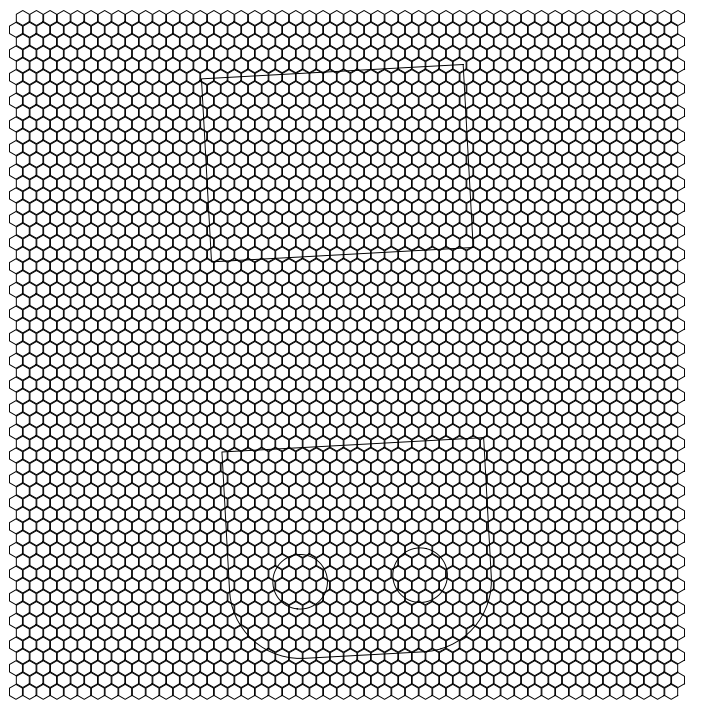Hexagon Island Boundaries generated across the entire region. The boundaries of the layer are shown, which are used for the intersection test.

The fully clipped scan strategy is shown below with the scanning ordered in the Y-direction.

## Conclusions

This post illustrates how one can effectively decompose a layer region into a series of repeatable ‘island’ units which can be processed in an efficient manner, by only clipping hatches at boundary regions. This potentially has the ability to define spatially aware island regions; for example this could be redefining island sizes or parameters towards the boundary of a part. It could be used to alter the scan strategies within the region too, with the effect of changing the thermal behavior.

The full excerpt of the example can be found on github at examples/example_custom_island_hatcher.py.

# PySLM: Exposure Map Generation & Visualisation

A curious feature was seeing the possibility to both generate and visualise the exposure points generated in along scan vectors. This also includes generating a pseudo ‘heatmap’ or exposure map of the energy deposited by the laser scanning across the surface. Variations of this idea, I have seen across a variety of commercial software – in particular Renishaw’s QuantAM, which is perhaps the source of inspiration for coming up with an approach.

Usually, there is not much indication as the relative exposure of energy from the laser into a layer based both the chosen laser parameters applied to the scan vectors of a specific LayerGeometry group and also the overall spatial distribution of scan vectors in a region. For instance, overlaps between scan vectors will in theory deposit more energy across time into a localised region . Ideally, the exposure of energy from the laser is very uniform across the regions.

It is debatable whether there is particular usefulness of visualising the exposure map of the laser – but in practice can be used to differentiate laser scan parameters used on models in parametric studies. With further thought, a cheap numerical simulation could potentially capture the transient heat distribution generated by the sequential deposition of these exposure points.

#### A Light Background on Laser Exposure:

Hopefully someone can further comment on of this and perhaps correct me…

SLM systems are built using fiber laser systems offering a very fine, high quality controlled laser beam. Two types of laser operation exist: continuous wave (CW) and pulsed.

In practice, with pulsed lasers used in AM platforms, the scan vector is decomposed across a number of exposure points at a set ‘pointDistance’ from each other along the scan vector. Each exposure irradiates energy into the powder-bed surface at a fixed energy (inferred from the average laserPower) for a set time period referred as a ‘pointExposureTime‘. After exposure, the galvo-mirrors instantaneously move to direct the beam to the next exposure point. The relative speed this occurs optically gives the illusion that the laser is continuously moving. Continuous wave, as the name describes, emits continuous irradiation whilst the beam traverses the surface. The laser speed parameter is used here.

Different systems use either pulsed, continuous, or even both modes of operation, offering different benefits. This in principle is the reason for BuildStyle class containing the following parameters to cover both situations:

• Point Exposure Time
• Point Distance
• Laser Speed
• Laser Power – used across both modes

Ultimately, this does not matter, but the proposed method obviously requires the user to set at minimum the pointDistance parameter in order for the following methodology to work.

#### Proposed Methodology

Taking an existing Layer containing LayerGeometry, the exposure points are generated individually for each scan vector. Given the potential number of scan vectors within a layer, it would be beneficial to perform this efficiently.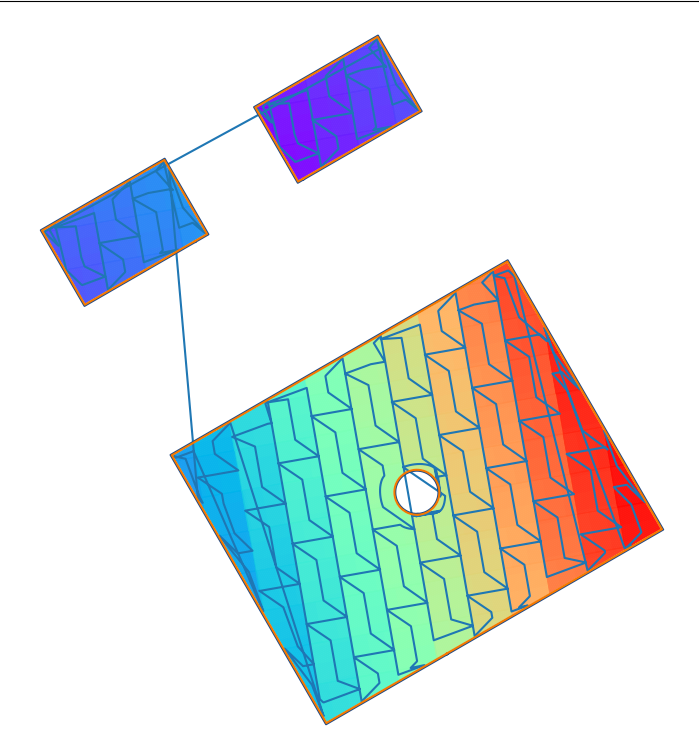Example part that is hatched with a series of overlapping 5mm island. The scan order is indicated by the blue line.

The basic process to do this is based on the following. The scan vector \textbf{v} has a particular length. Based on the point exposure distance, we can equally divide along this a number of points. Using both v_o and its direction ,we can then project a given number of exposure points along this line.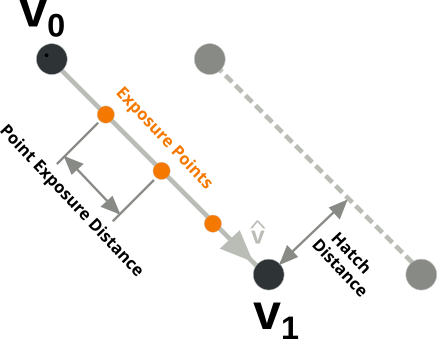Definition of a hath vector and decomposing each scan vector into a series of exposure points.

An attempt to do this more efficiently and exploit vectorisation built into numpy was achieved by the following procedure. The following properties of each scan vector (\textbf{v}) is obtained:

• start point (v_o)
• normalised scan vector direction (\hat{\textbf{v}})
• scan vector length \lVert \textbf{v} \rVert

For each LayerGeometry object, these are obtained across each scan vector as follows assuming that it is a HatchGeometry object:

# Calculate the length of the hatch vector and the direction
coords = layerGeom.coords.reshape(-1, 2, 2)
delta = np.diff(coords, axis=1).reshape(-1, 2)
lineDist = np.hypot(delta[:, 0], delta[:, 1]).reshape(-1, 1)

# Take the first coordinate
v0 = coords[:, 1, :].reshape(-1, 2)

# Normalise each scan vector direction
vhat = -1.0 * delta / lineDist

The number of point exposure across each vector is calculated by simple division. Unfortunately there is rounding, so the exposure may be missing at the end of the scan vector.

# Calculate the number of exposure points across the hatch vector based on its length
numPoints = np.ceil(lineDist / pointDistance).astype(np.int)


The total number of exposure points is calculated and is used to pre-populate some arrays, where the terms are used to extrapolate the exposure point distance (Line 16) are generated. Line 10 is key here and idxArray stores a range to account for each exposure point along the scan vector

Unfortunately, there isn’t a convenient way vectorise this so it is performed across each scan vector. Finally, the exposure points are generated by extrapolating them from v_o along \hat{\textbf{v}}.

# Pre-populate some arrays to extrapolate the exposure points from
totalPoints = int(np.sum(numPoints))
idxArray = np.zeros([totalPoints, 1])
pntsArray = np.zeros([totalPoints, 2])
dirArray = np.zeros([totalPoints, 2])

idx = 0
for i in range(len(numPoints)):
j = int(numPoints[i])
idxArray[idx:idx + j, 0] = np.arange(0, j)
pntsArray[idx:idx + j] = p0[i]
dirArray[idx:idx + j] = vhat[i]
idx += j

# Calculate the hatch exposure points
hatchExposurePoints = pntsArray + pointDistance * idxArray * dirArray

This generates a set of exposure points, which can be seen in the figure below:

Once the exposure points are generated, the deposited energy (laserPower x pointExposureTime) is added to each point and stored as a third column for later use.

#### Exposure Map Generation

Generating the exposure map is trivial. For a selected resolution chosen by the user, the bitmap slice is used currently to get the image to process with. The exposure coordinates are simply mapped, given an offset and resolution onto the image. The deposited energy is then summed accordingly across each pixel. The energy per area (latex]Q[/latex]), is calculated by diving by the aerial coverage of a single pixel.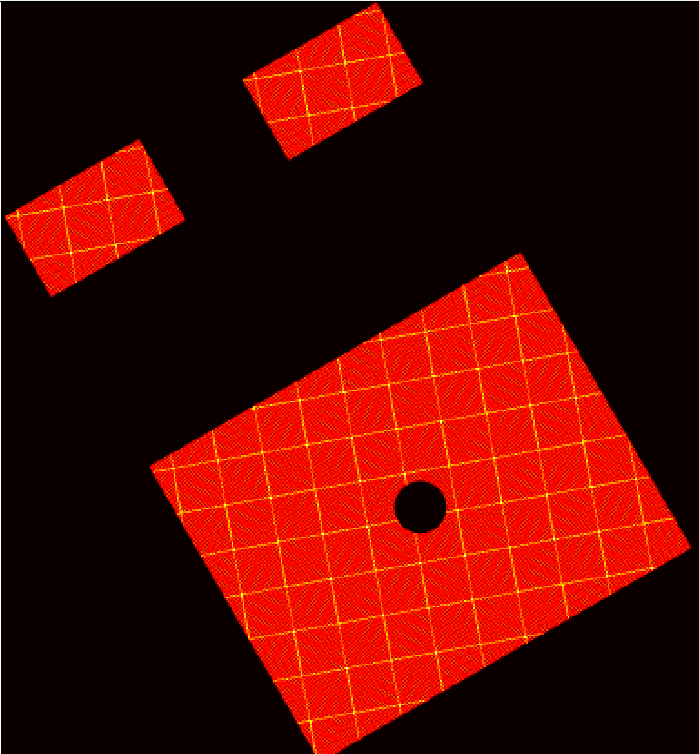Exposure / Heat Map showing the deposited energy applied to the position and laser parameters used. Notice the overlap region between islands show a higher energy deposition.

The resolution is arbitrarily selected. The above figure is generated with a resolution of 0.2 mm. It can be seen in this example that the there is a greater energy deposition across the the overlap regions between the islands. There are some ‘aliasing’ effects due to discretion onto a finer grid which is dependent on the resolution chosen.

## Conclusions

The above exposure map generation might have little scientific utility by itself. Rather it can offer a method to potentially visualise the energy deposition across a layer.

However, many thermo-mechanical AM build simulation that incorporate thermal effects in addition to the inherent strain approach could potentially incorporate a reference exposure map across the layer rather than assuming a single aerial heat-flux applied to the layer. This could actually be carried out across a ‘packet’ or number of layers to better correspond with the geometry and scan strategies employed.

## Documented Example

The code for this can be found in examples/example_heatmap.py on the github repository.

# Multi-threading Slicing & Hatching in PySLM

In PySLM, the slicing and hatching problem is inherently parallelisable simply because we can discretise areas the geometry into disrcete layers that for most situations behave independent. However, the actual underlying algorithms for slicing, offsetting the boundaries, clipping the hatch vectors is serial (single threaded). In order to significantly reduce the process time, multi-threaded processing is very desirable

Unfortunately, Python like most scripting or interpreter languages of the past are not inherently designed or destined to be multi-threaded. Perhaps, this may change in the future, but other scripting languages may fill this computational void (Rust, Julia, Go). Python, by intentions limits any multi-threaded use in scripts by using a construct known as the GIL – Global Interpreter Lock. This is a shared situation in other common scripting languages Matlab (ParPool), Javascript (Worker) where the parallel computing capability of multi-core CPUs cannot be exploited in a straightforward manner.

To some extent special distributions such as via the Anaconda distribution, core processing libraries such as numpy, scipy, scikit libraries internally are generally multi-threaded and support vectorisation via native CPU extensions. More computational mathematical operations and algorithms can to some extent be optimised to run in parallel automatically using numba, numexpr, and however, this cannot cover more broad multi-functional algorithms, such as those used in PySLM.

Python has the thread module for multi-threaded processing, however, for CPU bound processing it has very limited use This is because Python uses the global interpreter lock – GIL and this only allows one programming thread (i.e. one line) to be executed at any instance. It is mainly used for asynchronous IO (network or filesystem) operations which can be processed in the background.

#### Use of Multiprocessing Library in PySLM

The other option is to use the multiprocessing library built into the core Python distribution. Without going into too much of the formalities, multi-processing spawns multiple python processes and assign batches of work. The following programming article I found as a useful reference to the pitfalls of using the library.

In this implementation, the Pool and Manager modules are used to more optimally process the geometry. The most important section is to initialise the multiprocessing library with the ‘spawn‘ method, which stops random interruptions during the operation as discussed in the previous article.

from multiprocessing import Manager
from multiprocessing.pool import Pool
from multiprocessing import set_start_method

set_start_method("spawn")

The Manager.dict acts as a ‘proxy‘ object used to more efficiently store data which is shared between each process that is launched. Without using manager, for each process launch, a copy of the objects passed are made. This is important for the geometry or Part object, which if it were to contain a lattice of a set ofs complex surface would become expensive to copy.

d = Manager().dict()
d['part'] = solidPart
d['layerThickness'] = layerThickness # [mm]


A Pool object is used to create a set number of processes via setting the parameter processes=8 (typically one per CPU core). This is a fixed number re-used across a batch through the entire computation which removes the cost of additional copying and initialising many process instances. A series of z slice levels are created representing the layer z-id. These are then merged into a list of tuple pairs with the Manager dictionary and is stored in processList.

Pool.map is used to perform the slice function (calculateLayer) and collect all computed layers following the computation.

p = Pool(processes=8)

numLayers = solidPart.boundingBox / layerThickness
z = np.arange(0, numLayers).tolist()

processList = list(zip([d] * len(z), z))

# Run the pro
layers = p.map(calculateLayer, processList)

The slicing function is fairly straightforward and just unpacks the arguments and performs the slicing and hatching operation. Note: each layer needs to currently initialise its own instance of a Hatcher class because this is not shared across all the processes. This carries a small cost, but means each layer can process entirely independently; in this example the change is the hatchAngle across layers. The layer position is calculated using the layer position (zid) and layerThickness.

def calculateLayer(input):
# Typically the hatch angle is globally rotated per layer by usually 66.7 degrees per layer
d = input
zid= input

layerThickness = d['layerThickness']
solidPart = d['part']

# Create a StripeHatcher object for performing any hatching operations
myHatcher = hatching.Hatcher()

# Set the base hatching parameters which are generated within Hatcher
layerAngleOffset = 66.7
myHatcher.hatchAngle = 10 + zid * 66.7
myHatcher.volumeOffsetHatch = 0.08
myHatcher.spotCompensation = 0.06
myHatcher.numInnerContours = 2
myHatcher.numOuterContours = 1
myHatcher.hatchSortMethod = hatching.AlternateSort()

#myHatcher.hatchAngle += 10

# Slice the boundary
geomSlice = solidPart.getVectorSlice(zid*layerThickness)

# Hatch the boundary using myHatcher
layer = myHatcher.hatch(geomSlice)

# The layer height is set in integer increment of microns to ensure no rounding error during manufacturing
layer.z = int(zid*layerThickness * 1000)
layer.layerId = int(zid)

return zid

The final step to use multiprocessing in Python is the inclusion of the python __name__ guard i.e:

if __name__ == '__main__':
main()

The above is unfortunate because it makes debugging slightly more tedious in editors, but is the price for extra performance.

### Performance Improvement

The performance improvement using the multiprocesssing library is shown in the table below for a modest 4 core laptop (my budget doesn’t stretch that far).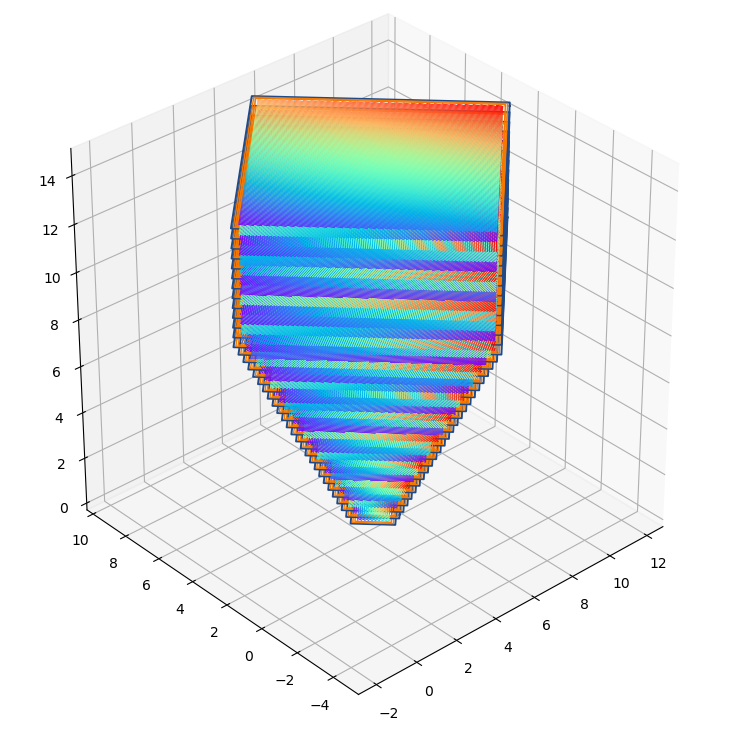Matplotlib Figure showing every subsequent 10 layers of hatching for the geometry but is shown reduced scale.

This was performed on the examples/inversePyramid.stl geometry with an overall bounding box size [90 \times 90 \times 60] mm, hatch distance h_d=0.08mm and the layer thickness set at 40 μm.

Given these are approximate timings, it is nearly linear performance improvement for the simple example. However, it can be seen choosing more processes beyond cores, does squeeze some extra performance out – perhaps due to Intel’s hyperthreading. Below shows that the CPU is fully utilised.

### Conclusions:

This post shows how one can adapt existing routines to generate multi-processing slicing and hatching with PySLM. In the future, it is desirable to explore a more integrated class structure for hooking functions onto. Other areas that are of interest to explore are potentially the use of GPU computing to parallelise some of the fundamental algorithms.

### Example

The example showing this that can be run is example_3d_multithread.py in the github repo.